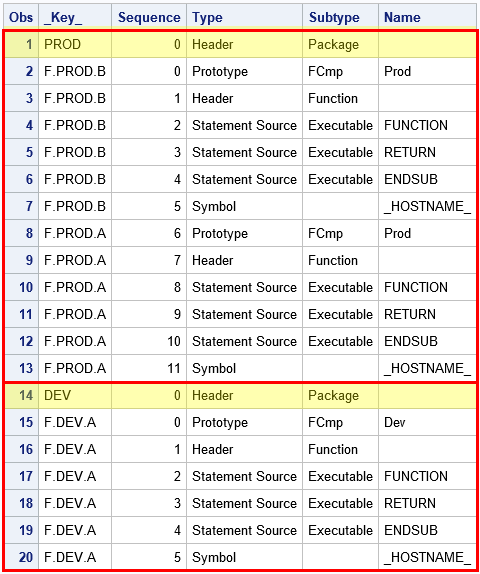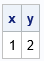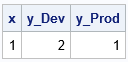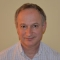I got a lot of feedback about my recent article about how to find roots of nonlinear functions by using the SOLVE function in PROC FCMP. A colleague asked how the FCMP procedure stores the functions. Specifically, why the OUTLIB= option on the PROC FCMP statement use a three-level syntax: OUTLIB=libref.DataSetName.PackageName. The three levels are a libref, a data set name, and a package name. The documentation is terse about what the third level (the package name) is used for and why it is important. This article describes how the FCMP-defined functions are stored, and how you can use the package name to call different versions of a function.

### How FCMP functions are stored

I started writing about the capabilities of the FCMP procedure in 2012, but the procedure itself goes back to SAS 9.2. Modern versions of SAS store functions in an analytic store (which is read by using PROC ASTORE) or in an item store (which is read by using PROC PLM). But these binary storage formats had not yet been developed back in the pre-9.2 days. So PROC FCMP stores functions in a SAS data set. That means you can use PROC PRINT to investigate how PROC FCMP stores functions.

When you use the OUTLIB= option in PROC FCMP, you specify a three-level name: OUTLIB=libref.DataSetName.PackageName. The first two levels specify the name of a SAS data set. This data set is created if it doesn't exist, or it is modified if it already exists. The third level is used as a text field in a variable named _KEY_, which enables one data set to contain functions that belong to different packages. The package name becomes important if two packages define a function that has the same name.

To demonstrate, let's define some functions and store them in a data set named Work.MyFuncs. The following statements create two functions (A and B) that belong to the 'PROD' (for 'Production') package and one function (A) that belongs to the 'DEV' (for 'Development') package. Notice that both packages have a function named 'A'. The following statements define the functions and use PROC PRINT to display a portion of the Work.MyFuncs data set:

```/* Store all functions in the data set WORK.MyFuncs */ /* Define functions in 'PROD' package */ proc fcmp outlib=work.MyFuncs.Prod; function A(x); return( x ); /* in the 'Prod' pkg, A(x) equals x */ endsub; function B(x); return( x > 0 ); endsub; quit;   /* Define functions in 'DEV' package */ proc fcmp outlib=work.MyFuncs.Dev; function A(x); return( 2*x ); /* the 'Dev' pkg uses a different definition for A(x) */ endsub; quit;   proc print data=work.MyFuncs; var _Key_ Sequence Type Subtype Name; run;```The output from PROC PRINT is shown. The data set contains 20 rows. I have put a red rectangle around rows 1–13 and another around rows 14–20. Each rectangle defines a package. The names of the packages are defined by the observations where Subtype='Package', which are highlighted in yellow. The Type, Subtype, and Name columns indicate how the FCMP statements that define the functions are stored in the data set. The _KEY_ column identifies which rows define which functions. There are other columns (not shown) that store the actual content of each function.

This output shows how the third level of the OUTLIB= option is used. The _KEY_ column records the package name and appends each function name ('A' or 'B') to the name of the package. So PROC FCMP knows that there are three stored functions whose full names are PROD.A, PROD.B, and DEV.A.

### Calling a function from the DATA step

Since there are two functions called 'A', what happens if I call 'A' from a SAS DATA step? The answer is that the DATA step uses the most recent definition, which in this example is DEV.A. To alert you to the fact that calling 'A' is ambiguous, the SAS log displays a warning. You can also use the _DISPLAYLOC_ flag on the CMPLIB= system option to display the origin of each call to an FCMP function, as follows:

```/* Tell the DATA step where to look for unresolved functions. The _DISPLAYLOC_ flag shows the full name for each call to an FCMP function */ options cmplib=(work.MyFuncs _DISPLAYLOC_); data Want; x = 1; y = A(x); /* y is the result of the latest definition */ run;   proc print data=Want noobs; run;```
```WARNING: Function 'A' was defined in a previous package. 'A' in current package DEV will be used as default when the package name is not specified.   NOTE: Function 'A' loaded from work.MyFuncs.DEV.```The value of the X and Y variables make it clear that the function DEV.A was called (because A(x)=2*x in that definition). The WARNING and NOTE in the SAS log reinforce this fact.

### Choosing which package to call

The WARNING in the previous section says that the current (most recent) package "will be used as default when the package name is not specified." This message seems to imply that you can somehow call PROD.A, which is the other stored function that is named 'A'. This is, in fact, true. The PROC FCMP documentation states, "to select a specific subroutine when there is ambiguity, use the package name and a period as the prefix to the subroutine name."

You cannot specify the package name directly in the DATA step, but you can specify the package name in an FCMP function. So, for example, you can define a function called 'ChooseA' that includes a flag that indicates which package to use. The following PROC FCMP statements define a function that will call either PROD.A or DEV.A, depending on the value of a parameter. This wrapper function can then be called in the DATA step:

```/* In PROC FCMP, you can "dis-ambiguate" by using a two-level function name */ proc fcmp outlib=work.MyFuncs.Choose; function ChooseA(x, choice \$); if upcase(choice)="DEV" then return( Dev.A(x) ); else return( Prod.A(x) ); endsub; quit;   data WantChoice; x = 1; y_Dev = ChooseA(x, "Dev"); /* call Dev.A */ y_Prod = ChooseA(x, "Prod"); /* call Prod.A */ run;   proc print data=WantChoice noobs; run;```From the definitions of DEV.A and PROD.A, you can verify that each function was called correctly. Because the _DISPLAYLOC_ option is still active, the SAS log also indicates that each function was called.

### Summary

This article was motivated by a question about how the FCMP procedure stores functions. The answer is that the OUTLIB= option on the PROC FCMP statement requires a libref, a data set name, and a package name. In most circumstances, you do not need to use the package name. The package name becomes important only if two different packages each support a function that has the same name. In that case, you can use the package name to disambiguate the function call.

Personally, I prefer to avoid having two packages that define the same function, but if you cannot avoid it, this trick shows you how to handle it. Eberhardt (2009, p. 15) discusses a related issue, which is how to call functions that are stored in two (or more) different data sets.

ShareDistinguished Researcher in Computational Statistics

Rick Wicklin, PhD, is a distinguished researcher in computational statistics at SAS and is a principal developer of SAS/IML software. His areas of expertise include computational statistics, simulation, statistical graphics, and modern methods in statistical data analysis. Rick is author of the books Statistical Programming with SAS/IML Software and Simulating Data with SAS.

1.Thank you, Rick, for this very clear and thorough explanation.

Now it is not a mystery anymore. Now, I clearly see a disconnect between defining and storing a function as a three-level entity (outlib=libref.dataset.package) and inability to reference that 3-level entity when using a function (options cmplib=libref.dataset;) in a data step. The wrapper solution when functions can be referenced by package.funcname within another PROC FCMP is valid but hardly efficient as it creates another layer of complexity and makes data step code environment-dependent (you can't simply migrate code y_Dev = ChooseA(x, "Dev"); from Development to Production without having to modify the code to code y_Dev = ChooseA(x, "Prod"). I think the better practice would be either to store function versions in different data sets (libref.prod_func, libref.dev_func) or in different libraries (prodlib.func, devlib.func) and not rely on packages at all. I would consider them as "vestigial" elements of the PROC FCMP evolution and always name them as "_" (or any other valid special name) just to satisfy the outlib= syntax (e.g. outlib=prodlib.myfunctions._ ).

•I agree with much of what you say, especially about preferring data set names over package names. However, as mentioned in the post, SAS programmers can call FCMP function from some procedures, and my understanding is that you can use the package name directly in the procedure. Therefore the package name is more useful in that context.

•Bartosz Jablonski on

I think it would be good "SASware Ballot Idea" to extend data step's behaviour to allow for use the third level with a "::" or "#" or other relevant symbol, like: ```y = prod::function(x);``` or ```y = dev#function(x);``` :-) or even with just the dot notation: ```y = prod.function(x);``` compiler would only had to check if it isn't a hash table ;-)

In the SAS Packages Framework (https://github.com/yabwon/SAS_PACKAGES) the third level is by default set to "package".

All the best
Bart

2.This is great! I had always wondered about that third-level name. Thanks for putting this together.